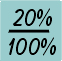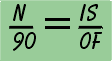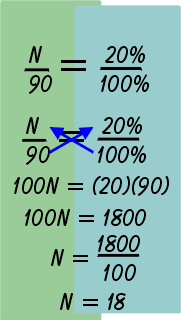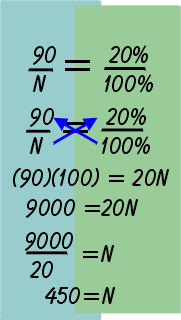What is 20% of 90? Wecan solve this problem by using two proportions. The first proportion will be the percent we are looking for: 20% or 20/100. The second proportion will have the number we are looking for, or N, as a numerator and the whole number 90 as a denominator: N/90To solve the problem, we cross multiply.15% of the members of the football team play both offense and defense. The Spartans have 60 players on their squad. How many players play both offense and defense?20% of what number is 90? The first proportion we use is 20% or 20/100. The second proportion will have the whole number 90 as a numerator and the number we are looking for, or N, as the denominator. To solve the problem, we cross multiply. 20 x N = 90 x 100 20N = 9000 N = 450 Therefore the number we are looking for is 450.

Calculating Simple Interest

 writer:Kevin Gallagher

Percent